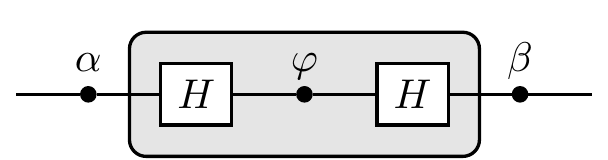## 5.4 Back to qubits

Let’s see how this formalism works for qubits. The n-fold tensor product of vectors from the standard basis \{|0\rangle,|1\rangle\} represent binary strings of length n. For example, for n=3, \begin{aligned} |0\rangle\otimes|1\rangle\otimes|1\rangle & \equiv |011\rangle \\|1\rangle\otimes|1\rangle\otimes|1\rangle & \equiv |111\rangle. \end{aligned} A classical register (that is, a collection of bits) composed of three bits can store only one of these two binary strings at any time; a quantum register composed of three qubits can store both of them in a superposition.

Indeed, if we start with the state |011\rangle and apply the Hadamard gate to the first qubit (which is the same as applying H\otimes\mathbf{1}\otimes\mathbf{1}), then, given that linear combinations distribute over tensor products, we obtain \begin{aligned} |011\rangle \overset{H\otimes\mathbf{1}\otimes\mathbf{1}}{\longmapsto} &\frac{1}{\sqrt{2}} \big(|0\rangle + |1\rangle\big) \otimes|1\rangle\otimes|1\rangle \\= &\frac{1}{\sqrt{2}} \big(|011\rangle + |111\rangle\big). \end{aligned}

In fact, we can even prepare this register in a superposition of all eight possible binary strings of length 3 at once: if we apply the tensor product operation H\otimes H\otimes H to the state |0\rangle\otimes|0\rangle\otimes|0\rangle = |000\rangle then we getThe resulting state is exactly a superposition of all binary string of length 3, and can also be written as \frac{1}{\sqrt{2}} \big(|0\rangle + |1\rangle\big) \otimes \frac{1}{\sqrt{2}} \big(|0\rangle + |1\rangle\big) \otimes \frac{1}{\sqrt{2}} \big(|0\rangle + |1\rangle\big).

In general, the tensor product operation H^{\otimes n}, which means “apply the Hadamard gate to each of your n qubits”, is known as the Hadamard transform, and it maps product states to product states. Like the Hadamard gate in the typical quantum interference circuit, the Hadamard transform opens (and closes) multi-qubit interference.